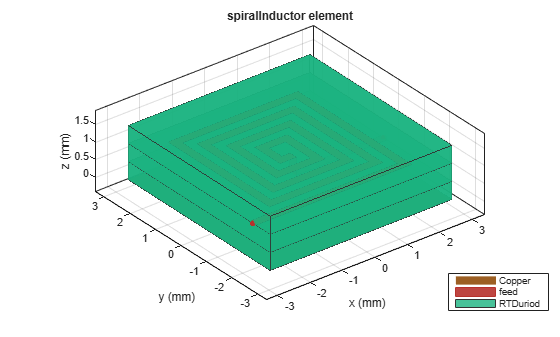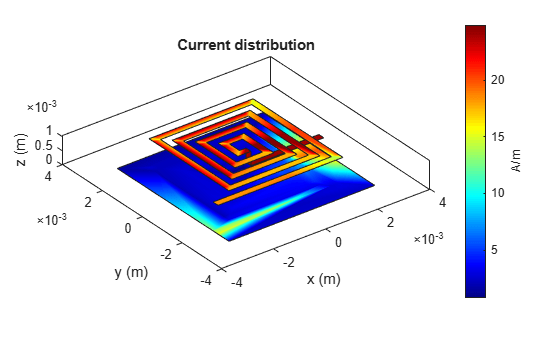# current

Calculate and plot current distribution

## Syntax

``current(rfpcbobject,frequency)``
``i = current(rfpcbobject,frequency)``
``[i,p] = current(rfpcbobject,frequency)``
``current(rfpcbobject,frequency,'dielectric')``
``i = current(rfpcbobject,frequency,'dielectric')``
``i = current(___,Name=Value)``

## Description

example

````current(rfpcbobject,frequency)` calculates and plots the absolute value of the current on the metal surface of a PCB component at the specified frequency.```
````i = current(rfpcbobject,frequency)` calculates the x, y, z components of the current on the surface of a PCB component at a specified frequencies.```

example

````[i,p] = current(rfpcbobject,frequency)` returns the current distribution and the points at which the current calculation was performed.```
````current(rfpcbobject,frequency,'dielectric')` calculates and plots the absolute value of the current at the specified frequency on the dielectric surface of the PCB component.```
````i = current(rfpcbobject,frequency,'dielectric')` calculates the x, y, z components of the current on the dielectric surface of a PCB component at the specified frequency.```
````i = current(___,Name=Value)` calculates the current on the surface of a PCB component using additional name-value arguments.```

## Examples

collapse all

Create a rat-race coupler with default properties.

`coupler = couplerRatrace;`

Set the excitation voltage and the phase angle at the ports of the coupler.

`v = voltagePort(4)`
```v = voltagePort with properties: NumPorts: 4 FeedVoltage: [1 0 0 0] FeedPhase: [0 0 0 0] PortImpedance: 50 ```
`v.FeedVoltage = [1 0 1 0]`
```v = voltagePort with properties: NumPorts: 4 FeedVoltage: [1 0 1 0] FeedPhase: [0 0 0 0] PortImpedance: 50 ```
`v.FeedPhase = [90 0 270 0]`
```v = voltagePort with properties: NumPorts: 4 FeedVoltage: [1 0 1 0] FeedPhase: [90 0 270 0] PortImpedance: 50 ```

Calculate and plot the current on the coupler at 3 GHz.

```figure current(coupler,3e9,Excitation=v)```Create a default spiral inductor.

```inductor = spiralInductor; show(inductor)```Calculate the current distribution on the inductor at 600 MHz.

`[i,p] = current(inductor,600e6)`
```i = 3×258 complex -0.0728 + 0.1411i 11.9623 -20.0648i -0.0003 + 0.0033i 0.1377 - 0.2742i -0.8881 + 1.3157i -0.4575 + 0.7285i 1.3875 - 2.2121i 12.0681 -16.3490i 0.7006 - 1.0115i 2.9532 - 4.1922i 0.2821 - 0.5294i 0.0013 - 0.0127i 1.6350 - 2.5088i 1.3896 - 2.2408i 2.8371 - 4.2355i 1.0830 - 1.5980i -0.3246 - 0.0169i 0.7000 - 1.0056i 3.8421 - 6.4594i 3.8406 - 6.4651i 0.1110 - 0.2372i 0.0209 - 0.1037i -1.2258 + 1.8863i -2.2446 + 3.1755i 2.6497 - 3.6287i 0.2039 - 0.2852i -1.7197 + 2.5320i 0.9097 - 1.4695i 3.4095 - 5.5835i 3.4082 - 5.5871i 0.0880 - 0.2120i 0.0776 - 0.1818i 0.0876 - 0.2143i 3.7547 - 6.1567i 0.0211 - 0.1012i -0.9996 + 1.3744i 0.6657 - 0.7463i 0.2874 - 0.4127i 0.2365 - 0.3316i 2.7579 - 4.2670i 1.5733 - 2.3511i 1.5981 - 2.3878i 2.6493 - 4.0647i 1.5727 - 2.3534i 2.7075 - 4.4861i 1.6105 - 2.4588i 0.1411 - 0.2887i 0.3393 - 0.5585i 0.1404 - 0.2911i -2.0990 + 3.0763i 0.7718 - 1.2051i 3.0732 - 4.7207i 0.8737 - 1.2552i 0.4853 - 0.4545i -0.0000 + 0.0016i -0.1933 + 0.2820i -0.5780 + 0.8052i 0.3758 - 0.4598i 2.1128 - 3.2166i 0.0004 - 0.0031i 0.5263 - 0.7310i -0.5784 + 0.8091i 0.5269 - 0.7354i 0.4922 - 0.7004i 0.4925 - 0.7033i 0.2004 - 0.2686i 0.3761 - 0.4621i 1.4634 - 2.2530i 2.2566 - 3.5057i 2.1080 - 3.1133i 1.5315 - 2.3127i 2.1633 - 3.2271i 0.0282 - 0.0192i 2.5126 - 3.3680i -2.3817 + 3.4310i -1.0066 + 1.6056i -0.0070 + 0.0243i 2.1777 - 3.1481i 2.4399 - 3.8930i 2.2005 - 3.2858i 1.7618 - 2.6811i 1.8160 - 2.8194i 1.8501 - 2.7291i 1.8527 - 2.7200i 2.2475 - 3.4213i 0.3649 - 0.2064i -1.3003 + 1.9099i -1.3622 + 2.1553i -1.8734 + 2.9418i 0.2778 - 0.3975i 1.3228 - 2.0330i 1.2748 - 1.9547i 0.2660 - 0.3727i 2.0023 - 2.9911i 1.7571 - 2.9126i 1.8873 - 2.6825i 1.8774 - 2.8611i 1.2849 - 2.0435i 1.7979 - 2.6871i -0.3638 + 0.4974i 0.0000 + 0.0000i 0.0000 + 0.0000i 0.0000 + 0.0000i 0.0000 + 0.0000i 0.0000 + 0.0000i 0.0000 + 0.0000i 0.0000 + 0.0000i 0.0000 + 0.0000i 0.0000 + 0.0000i 0.0000 + 0.0000i 0.0000 + 0.0000i 0.0000 + 0.0000i 0.0000 + 0.0000i 0.0000 + 0.0000i 0.0000 + 0.0000i 0.0000 + 0.0000i 0.0000 + 0.0000i 0.0000 + 0.0000i 0.0000 + 0.0000i 0.0000 + 0.0000i 0.0000 + 0.0000i 0.0000 + 0.0000i 0.0000 + 0.0000i 0.0000 + 0.0000i 0.0000 + 0.0000i 0.0000 + 0.0000i 0.0000 + 0.0000i 0.0000 + 0.0000i 0.0000 + 0.0000i 0.0000 + 0.0000i 0.0000 + 0.0000i 0.0000 + 0.0000i 0.0000 + 0.0000i 0.0000 + 0.0000i 0.0000 + 0.0000i 0.0000 + 0.0000i 0.0000 + 0.0000i 0.0000 + 0.0000i 0.0000 + 0.0000i 0.0000 + 0.0000i 0.0000 + 0.0000i 0.0000 + 0.0000i 0.0000 + 0.0000i 0.0000 + 0.0000i 0.0000 + 0.0000i 0.0000 + 0.0000i 0.0000 + 0.0000i 0.0000 + 0.0000i 0.0000 + 0.0000i 0.0000 + 0.0000i ```
```p = 3×258 0.0024 0.0025 0.0025 0.0023 -0.0001 -0.0022 -0.0009 -0.0019 0.0021 0 0.0016 -0.0019 0.0012 0.0006 0.0011 -0.0002 -0.0016 0.0019 0.0017 0.0018 0.0017 0.0016 0.0006 -0.0016 -0.0015 -0.0018 -0.0006 0.0011 0.0012 0.0013 0.0012 0.0011 0.0013 0.0014 0.0014 -0.0011 -0.0010 -0.0013 -0.0014 -0.0007 0.0007 0.0006 0.0001 0.0008 0.0007 0.0009 0.0007 0.0006 0.0008 0.0005 -0.0022 -0.0002 0.0017 0.0007 0.0027 0.0025 -0.0022 -0.0021 -0.0020 -0.0026 -0.0024 -0.0024 -0.0022 -0.0021 -0.0019 -0.0018 -0.0019 -0.0021 -0.0002 -0.0001 0.0012 0.0006 0.0021 0.0018 0.0018 0.0008 0.0022 -0.0012 -0.0002 -0.0002 0.0009 0.0004 0.0005 -0.0001 0.0011 0.0012 0.0013 -0.0002 0.0007 -0.0011 -0.0006 -0.0008 -0.0012 -0.0008 -0.0002 -0.0005 0.0006 0.0003 0.0003 0.0008 0 0 0 0 0 0 0 0 0 0 0 0 0 0 0 0 0 0 0 0 0 0 0 0 0 0 0 0 0 0 0 0 0 0 0 0 0 0 0 0 0 0 0 0 0 0 0 0 0 0 ```

Plot the current distribution.

`current(inductor,600e6)`## Input Arguments

collapse all

PCB component object, specified as an RF PCB object. For a complete list of the PCB components, microstrip bends, and traces, see PCB Components Catalog and Custom Geometry and PCB Fabrication.

Frequency to calculate the current distribution, specified as a scalar in Hz.

Example: 70e6

Data Types: `double`

### Name-Value Arguments

Example: `scale=log10`

Scale to visualize the current distribution on the surface of the PCB component, specified as a string or a character vector. The string values are either `'linear'`, `'log'`, or `'log10'` or as a function. You can specify any mathematical function such as `log`, `log10`, `cos`, or `sin`.

Data Types: `char` | `function_handle`

Excitation using as voltage source, specified function handle from the `voltagePort` function.

Data Types: `char` | `function_handle`

## Output Arguments

collapse all

x, y, z components of the current distribution, returned as a 3-by-n complex matrix in A/m. The value of the current is calculated on every triangle mesh on the surface of the PCB component.

Cartesian coordinates representing the center of each triangle in the mesh, returned as a 3-by-n real matrix.